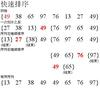1960年

C. A. R. Hoare

Pascal，c++等语言

quick sort

• 快速排序今天看到大神写的一篇快速排序的博客，肃然起敬，觉得原来快速排序这么简单 下面进行简单的试试快速排序的基本思想是 1、先从数列中取出一个数作为基准数 2、分区过程，将比这个数大的数全放到它的...
快速排序
【内推】日常实习和社招也可以简历发送到我邮箱，长期接受简历，部门做搜索产品研发，主要php和go语言！ 【2022百度提前批招聘】填写内推码可以免专业笔试，部门直接发起面试，有想去的部门可以发送简历到 927①56零36@qq.com 定向内推，本次招聘不影响正常校招流程，相当于多一次机会，赶紧填写内推码【am8eh4】试试吧！= = = = = = = = = = = = = = = = = = = = = = = = = = = = = = = = = = = = = = = = = = = = = = = = 今天看到大神写的一篇快速排序的博客，肃然起敬，觉得原来快速排序这么简单 下面进行简单的试试
快速排序的基本思想是

1、先从数列中取出一个数作为基准数

2、分区过程，将比这个数大的数全放到它的右边，小于或等于它的数全放到它的左边

3、再对左右区间重复第二步，直到各区间只有一个数

概括来说为 挖坑填数+分治法
下面举例来进行说明，主要有三个参数，i为区间的开始地址，j为区间的结束地址，X为当前的开始的值
第一步，i=0,j=9,X=21
01234567892132439854452346686
第二步，从j开始由，后向前找，找到比X小的第一个数a=4,此时i=0,j=6,X=21 进行替换
01234567894324398544523216686
第三步，由前往后找，找到比X大的第一个数a=32,此时i=2,j=6,X=21
01234567894214398544523326686
第四步，从j=6开始由，由后向前找，找到比X小的第一个数a=4,此时i=2,j=0,X=21，发现j<=i,所以第一回结束
可以发现21前面的数字都比21小，后面的数字都比21大 接下来对两个子区间[0,0]和[2,9]重复上面的操作即可
下面直接给出过程，就步详细解说了
i=2,j=6,X=43
01234567894214398544523326686
i=4,j=6,X=43
01234567894213298544523436686
i=4,j=5,x=43
01234567894213243544523986686
i=5,j=5,x=43
01234567894213223434554986686
然后被分为了两个子区间[2,3]和[5,9]
…最后排序下去就是最终的答案
01234567894212332434554668698
总结：

1．i =L; j = R; 将基准数挖出形成第一个坑a[i]。

2．j–由后向前找比它小的数，找到后挖出此数填前一个坑a[i]中。

3．i++由前向后找比它大的数，找到后也挖出此数填到前一个坑a[j]中。

4．再重复执行2，3二步，直到i==j，将基准数填入a[i]中。

代码
class Solution {
public void sort(int left,int right,int[] array) {
if (left>=right) return ;
int start=left;
int end=right;
int flag=left;
while(left<right) {
while ((left<right)&&(array[right]>=array[flag])) {
right--;
}
if (array[right]<array[flag]) {
int tmp=array[right];
array[right]=array[flag];
array[flag]=tmp;
flag=right;
}
while ((left<right)&&(array[left]<=array[flag])) {
left++;
}
if (array[left]>array[flag]) {
int tmp=array[left];
array[left]=array[flag];
array[flag]=tmp;
flag=left;
}
}
sort(start, left-1, array);
sort(left+1, end, array);
}
}

分享一个比较牛逼的学习java的网站，基础知识和架构等都有，连接如下： http://how2j.cn?p=54321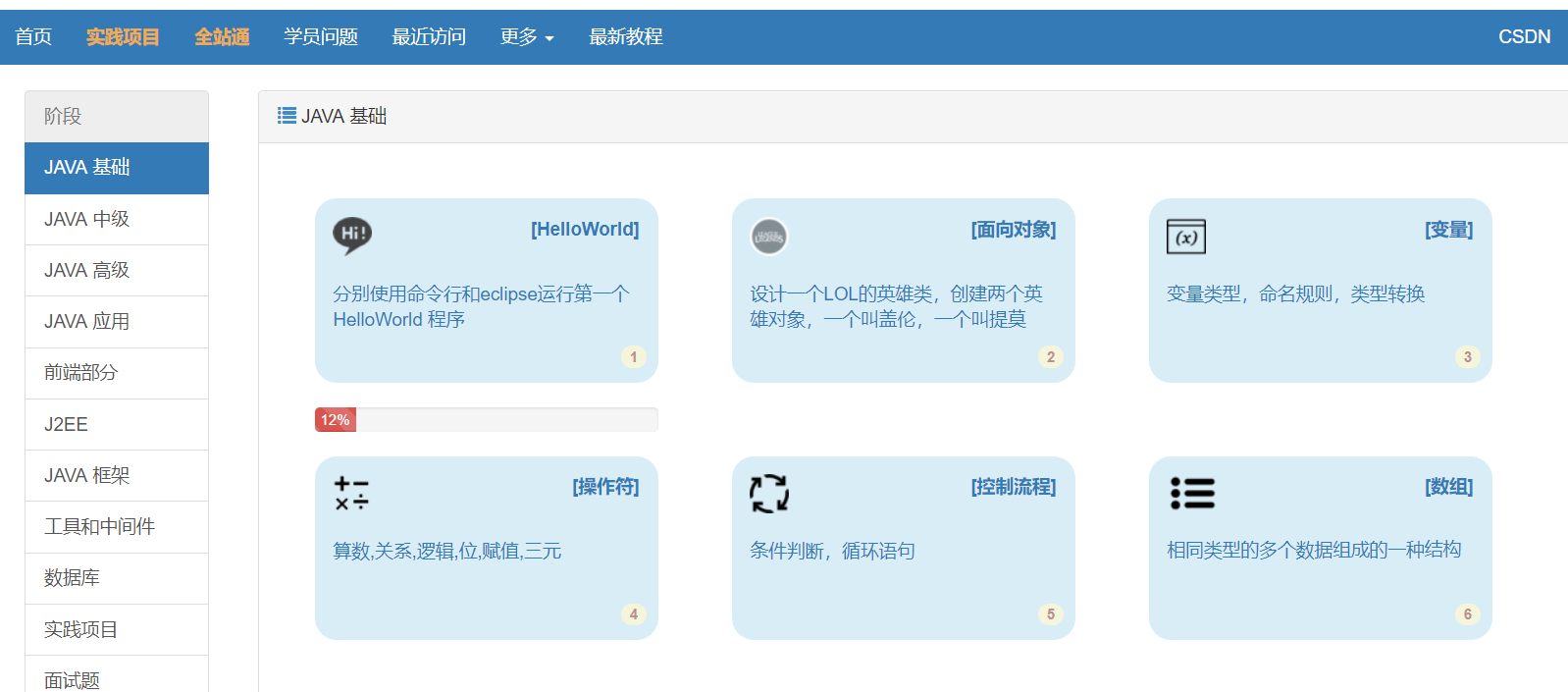展开全文• ## 快速排序

万次阅读 2020-04-24 01:10:08
文章目录快速排序算法排序流程动图算法分析java代码 快速排序算法 快速排序是一种分治的排序算法，和冒泡排序同属于交换排序。 快速排序在每一轮都挑选一个基准元素，并让比它小的元素移到数列的一边，比它大的元素...


文章目录
快速排序算法排序流程动图算法分析java代码

快速排序算法
快速排序是一种分治的排序算法，和冒泡排序同属于交换排序。
快速排序在每一轮都挑选一个基准元素，并让比它小的元素移到数列的一边，比它大的元素移到数列数列的另一边，从而把数列拆分成两部分。
排序流程
从数列中挑出一个基准元素（pivot）；所有比基准值小的元素移动到基准前面，其它比基准值大的元素移到基准的后面（相同的数可以不动）。完成后该基准就处于数列的中间位置，称为分区操作；递归地把小于基准值元素的子数列和大于基准值元素的子数列排序。
动图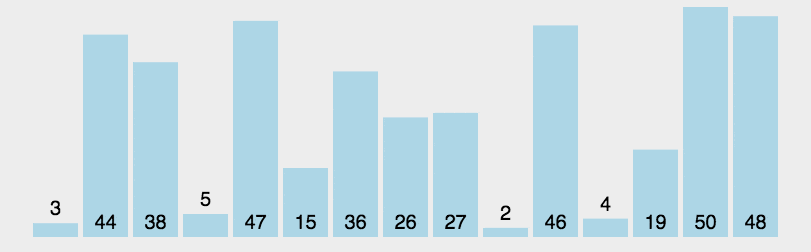算法分析
快速排序算法的效率在序列越乱的时候，效率越高，在数据有序时，会退化成冒泡排序。
优点：极快数据移动少。
缺点：不稳定。
最佳情况：O(nlogn)

最差情况：O(n^2)

平均情况：O(nlogn)

空间复杂度：O(nlogn)

java代码
@Override
public <T extends Comparable<T>> T[] sort(T[] array) {
doSort(array, 0, array.length - 1);
return array;
}

private static <T extends Comparable<T>> void doSort(T[] array, int left, int right) {
if (left < right) {
int pivot = randomPartition(array, left, right);
doSort(array, left, pivot - 1);
doSort(array, pivot, right);
}
}

private static <T extends Comparable<T>> int randomPartition(T[] array, int left, int right) {
int randomIndex = left + (int)(Math.random()*(right - left + 1));
swap(array, randomIndex, right);
return partition(array, left, right);
}

private static <T extends Comparable<T>> int partition(T[] array, int left, int right) {
int mid = (left + right) / 2;
T pivot = array[mid];

while (left <= right) {
while (less(array[left], pivot)) {
++left;
}
while (less(pivot, array[right])) {
--right;
}
if (left <= right) {
swap(array, left, right);
++left;
--right;
}
}
return left;
}

展开全文算法 排序算法 数据结构 堆排序
• ## 快速排序---(面试碰到过好几次)

万次阅读 多人点赞 2018-09-10 12:20:21
快速排序,说白了就是给基准数据找其正确索引位置的过程.    如下图所示,假设最开始的基准数据为数组第一个元素23,则首先用一个临时变量去存储基准数据,即tmp=23;然后分别从数组的两端扫描数组，设两个指示...
原理:
快速排序,说白了就是给基准数据找其正确索引位置的过程.    如下图所示,假设最开始的基准数据为数组第一个元素23,则首先用一个临时变量去存储基准数据,即tmp=23;然后分别从数组的两端扫描数组，设两个指示标志:low指向起始位置，high指向末尾.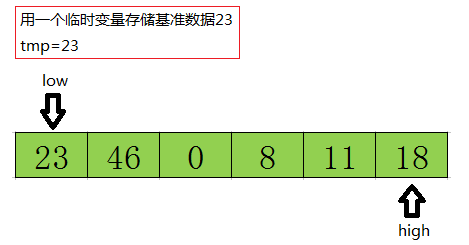首先从后半部分开始，如果扫描到的值大于基准数据就让high减1,如果发现有元素比该基准数据的值小(如上图中18<=tmp)，就将high位置的值赋值给low位置 ,结果如下: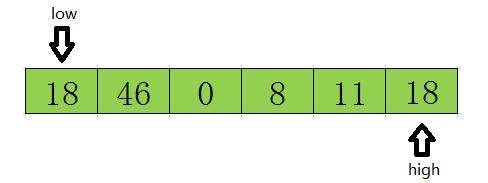然后开始从前往后扫描,如果扫描到的值小于基准数据就让low加1,如果发现有元素大于基准数据的值(如上图46=>tmp)，就再将low位置的值赋值给high位置的值,指针移动并且数据交换后的结果如下: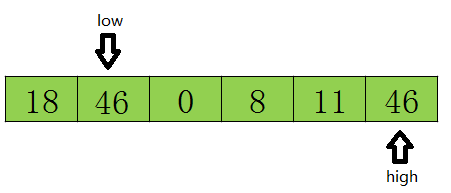然后再开始从后向前扫描,原理同上,发现上图11<=tmp,则将low位置的值赋值给high位置的值 ,结果如下: 然后再开始从后向前扫描,原理同上,发现上图11<=tmp,则将high位置的值赋值给low位置的值,结果如下: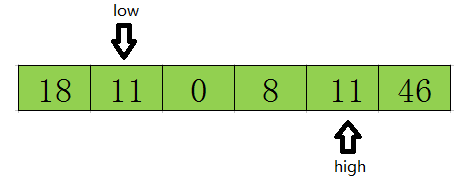然后再开始从前往后遍历,直到low=high结束循环,此时low或high的下标就是基准数据23在该数组中的正确索引位置.如下图所示.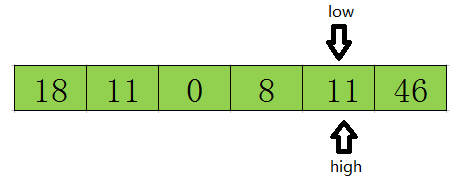这样一遍走下来,可以很清楚的知道,其实快速排序的本质就是把基准数大的都放在基准数的右边,把比基准数小的放在基准数的左边,这样就找到了该数据在数组中的正确位置.   以后采用递归的方式分别对前半部分和后半部分排序，当前半部分和后半部分均有序时该数组就自然有序了。
一些小结论
从上面的过程中可以看到:
①先从队尾开始向前扫描且当low < high时,如果a[high] > tmp,则high–,但如果a[high] < tmp,则将high的值赋值给low,即arr[low] = a[high],同时要转换数组扫描的方式,即需要从队首开始向队尾进行扫描了   ②同理,当从队首开始向队尾进行扫描时,如果a[low] < tmp,则low++,但如果a[low] > tmp了,则就需要将low位置的值赋值给high位置,即arr[low] = arr[high],同时将数组扫描方式换为由队尾向队首进行扫描.   ③不断重复①和②,知道low>=high时(其实是low=high),low或high的位置就是该基准数据在数组中的正确索引位置.
按照上诉理论我写的代码如下:
package com.nrsc.sort;

public class QuickSort {
public static void main(String[] args) {
int[] arr = { 49, 38, 65, 97, 23, 22, 76, 1, 5, 8, 2, 0, -1, 22 };
quickSort(arr, 0, arr.length - 1);
System.out.println("排序后:");
for (int i : arr) {
System.out.println(i);
}
}

private static void quickSort(int[] arr, int low, int high) {

if (low < high) {
// 找寻基准数据的正确索引
int index = getIndex(arr, low, high);

// 进行迭代对index之前和之后的数组进行相同的操作使整个数组变成有序
//quickSort(arr, 0, index - 1); 之前的版本，这种姿势有很大的性能问题，谢谢大家的建议
quickSort(arr, low, index - 1);
quickSort(arr, index + 1, high);
}

}

private static int getIndex(int[] arr, int low, int high) {
// 基准数据
int tmp = arr[low];
while (low < high) {
// 当队尾的元素大于等于基准数据时,向前挪动high指针
while (low < high && arr[high] >= tmp) {
high--;
}
// 如果队尾元素小于tmp了,需要将其赋值给low
arr[low] = arr[high];
// 当队首元素小于等于tmp时,向前挪动low指针
while (low < high && arr[low] <= tmp) {
low++;
}
// 当队首元素大于tmp时,需要将其赋值给high
arr[high] = arr[low];

}
// 跳出循环时low和high相等,此时的low或high就是tmp的正确索引位置
// 由原理部分可以很清楚的知道low位置的值并不是tmp,所以需要将tmp赋值给arr[low]
arr[low] = tmp;
return low; // 返回tmp的正确位置
}
}


展开全文• ## 快速排序算法——C/C++

万次阅读 多人点赞 2019-06-12 22:55:14
快速排序 1、算法思想 快速排序的基本思想：通过一趟排序将待排记录分隔成独立的两部分，其中一部分记录的关键字均比另一部分的关键字小，则可分别对这两部分记录继续进行排序，以达到整个序列有序。 2、实现原理 ...
快速排序
1. 算法思想
快速排序的基本思想：通过一趟排序将待排记录分隔成独立的两部分，其中一部分记录的关键字均比另一部分的关键字小，则可分别对这两部分记录继续进行排序，以达到整个序列有序。
2. 实现原理
2.1、设置两个变量 low、high，排序开始时：low=0，high=size-1。 2.2、整个数组找基准正确位置，所有元素比基准值小的摆放在基准前面，所有元素比基准值大的摆在基准的后面
默认数组的第一个数为基准数据，赋值给key，即key=array[low]。因为默认数组的第一个数为基准，所以从后面开始向前搜索（high–），找到第一个小于key的array[high]，就将 array[high] 赋给 array[low]，即 array[low] = array[high]。（循环条件是 array[high] >= key；结束时 array[high] < key）此时从前面开始向后搜索（low++），找到第一个大于key的array[low]，就将 array[low] 赋给 array[high]，即 array[high] = array[low]。（循环条件是 array[low] <= key；结束时 array[low] > key）循环 2-3 步骤，直到 low=high，该位置就是基准位置。把基准数据赋给当前位置。
2.3、第一趟找到的基准位置，作为下一趟的分界点。 2.4、递归调用（recursive）分界点前和分界点后的子数组排序，重复2.2、2.3、2.4的步骤。 2.5、最终就会得到排序好的数组。
3. 动态演示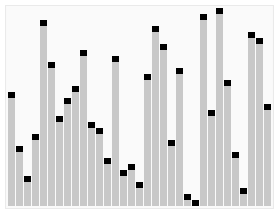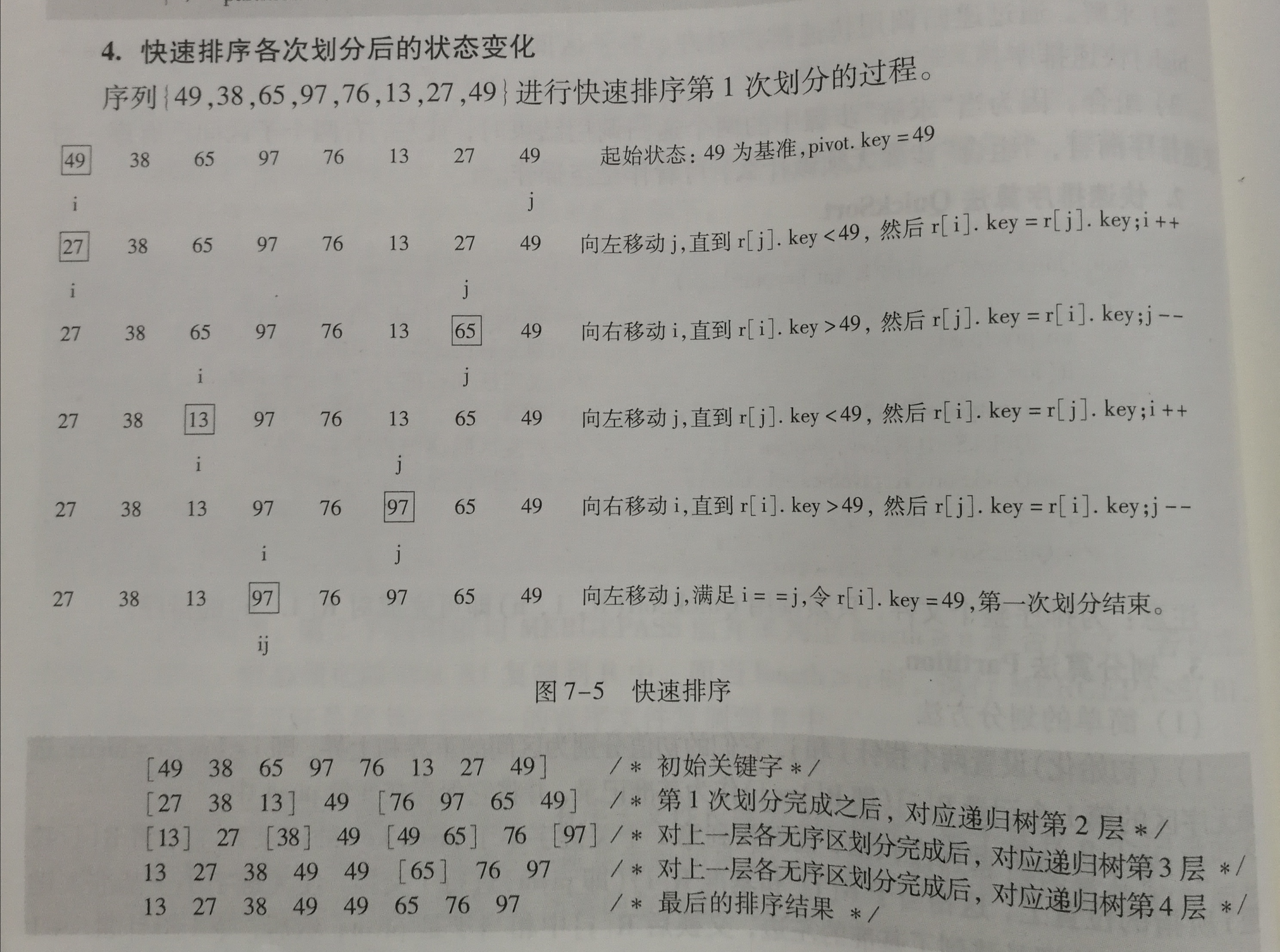4. 完整代码
三个函数 基准插入函数：int getStandard(int array[],int low,int high) （返回基准位置下标） 递归排序函数：void quickSort(int array[],int low,int high) 主函数：int main()
#include <stdio.h>
#include <stdlib.h>

void display(int* array, int size) {
for (int i = 0; i < size; i++) {
printf("%d ", array[i]);
}
printf("\n");
}

int getStandard(int array[], int i, int j) {
// 基准数据
int key = array[i];
while (i < j) {
// 因为默认基准是从左边开始，所以从右边开始比较
// 当队尾的元素大于等于基准数据 时,就一直向前挪动 j 指针
while (i < j && array[j] >= key) {
j--;
}
// 当找到比 array[i] 小的时，就把后面的值 array[j] 赋给它
if (i < j) {
array[i] = array[j];
}
// 当队首元素小于等于基准数据 时,就一直向后挪动 i 指针
while (i < j && array[i] <= key) {
i++;
}
// 当找到比 array[j] 大的时，就把前面的值 array[i] 赋给它
if (i < j) {
array[j] = array[i];
}
}
// 跳出循环时 i 和 j 相等,此时的 i 或 j 就是 key 的正确索引位置
// 把基准数据赋给正确位置
array[i] = key;
return i;
}

void QuickSort(int array[], int low, int high) {
// 开始默认基准为 low
if (low < high) {
// 分段位置下标
int standard = getStandard(array, low, high);
// 递归调用排序
// 左边排序
QuickSort(array, low, standard - 1);
// 右边排序
QuickSort(array, standard + 1, high);
}
}

// 合并到一起快速排序
// void QuickSort(int array[], int low, int high) {
//     if (low < high) {
//         int i   = low;
//         int j   = high;
//         int key = array[i];
//         while (i < j) {
//             while (i < j && array[j] >= key) {
//                 j--;
//             }
//             if (i < j) {
//                 array[i] = array[j];
//             }
//             while (i < j && array[i] <= key) {
//                 i++;
//             }
//             if (i < j) {
//                 array[j] = array[i];
//             }
//         }
//         array[i] = key;
//         QuickSort(array, low, i - 1);
//         QuickSort(array, i + 1, high);
//     }
// }

int main() {
int array[] = {49, 38, 65, 97, 76, 13, 27, 49, 10};
int size    = sizeof(array) / sizeof(int);

// 打印数据
printf("%d \n", size);
QuickSort(array, 0, size - 1);
display(array, size);

// int size      = 20;
// int array = {0};                 // 数组初始化
// for (int i = 0; i < 10; i++) {       // 数组个数
//     for (int j = 0; j < size; j++) { // 数组大小
//         array[j] = rand() % 1000;    // 随机生成数大小 0~999
//     }
//     printf("原来的数组：");
//     display(array, size);
//     QuickSort(array, 0, size - 1);
//     printf("排序后数组：");
//     display(array, size);
//     printf("\n");
// }

return 0;
}

5. 结果展示
（递归调用，不好展示每次排序结果）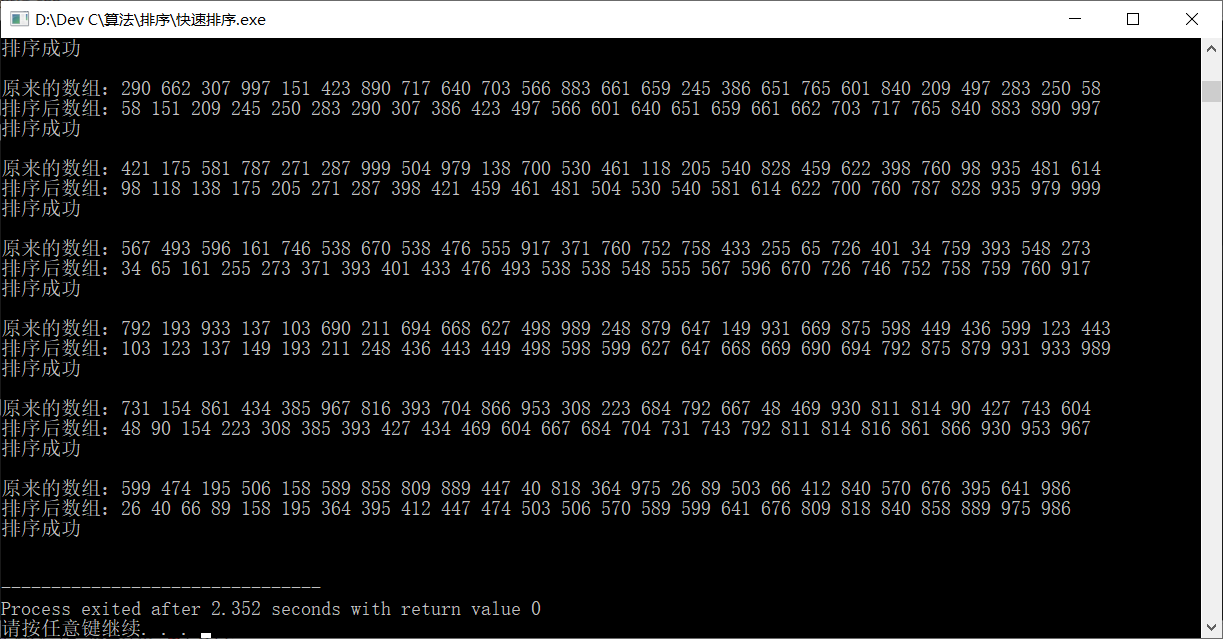6. 算法分析
时间复杂度：
最好：

O

(

n

l

o

g

2

n

)

O(n log_{2} n)

最坏：

O

(

n

2

)

O(n^2)

平均：

O

(

n

l

o

g

2

n

)

O(n log_{2} n)

空间复杂度：

O

(

n

l

o

g

2

n

)

O(n log_{2} n)

稳定性：不稳定
展开全文C/C++
• ## 快速排序（过程图解）

万次阅读 多人点赞 2018-07-02 12:10:50
假设我们现在对“612 79345 108”这个10个数进行排序。首先在这个序列中随便找一个数作为基准数（不要被这个名词吓到了，就是一个用来参照的数，待会你就知道它用来做啥的了）。为了方便，就让第一个数6作为基准数吧...
• ## 快速排序法（详解）

万次阅读 多人点赞 2019-07-01 17:27:49
假设对以下10个数进行快速排序： 6 1 2 7 9 3 4 5 10 8 我们先模拟快速排序的过程：首先，在这个序列中随便找一个数作为基准数，通常为了方便，以第一个数作为基准数。 6 1 2 ...
• 快速排序由于排序效率在同为O(N*logN)的几种排序方法中效率较高，因此经常被采用，再加上快速排序思想----分治法也确实实用，因此很多软件公司的笔试面试，包括像腾讯，微软等知名IT公司都喜欢考这个，还有大大小的...微软 算法 腾讯
• ## 算法 - 快速排序（C#）

万次阅读 多人点赞 2019-01-29 20:29:11
分享一个大牛的人工智能教程。零基础！通俗易懂！风趣幽默！希望你也加入到人工智能的队伍中来！... /* * 快速排序（QuickSort）是对冒泡排序的一种... * 然后再按此方法对这两部分数据分别进行快速排序，整个排序...算法 C#
• ## 快速排序算法

万次阅读 多人点赞 2019-01-11 21:09:08
但是这种算法时间复杂度高，当需要排序的元素较多时，程序运行时间很长，因此产生了快速排序算法。该算法的实现可分为以下几步： 1. 在数组中选一个基准数（通常为数组第一个）； 2. 将数组中小于基准数的数据移到......